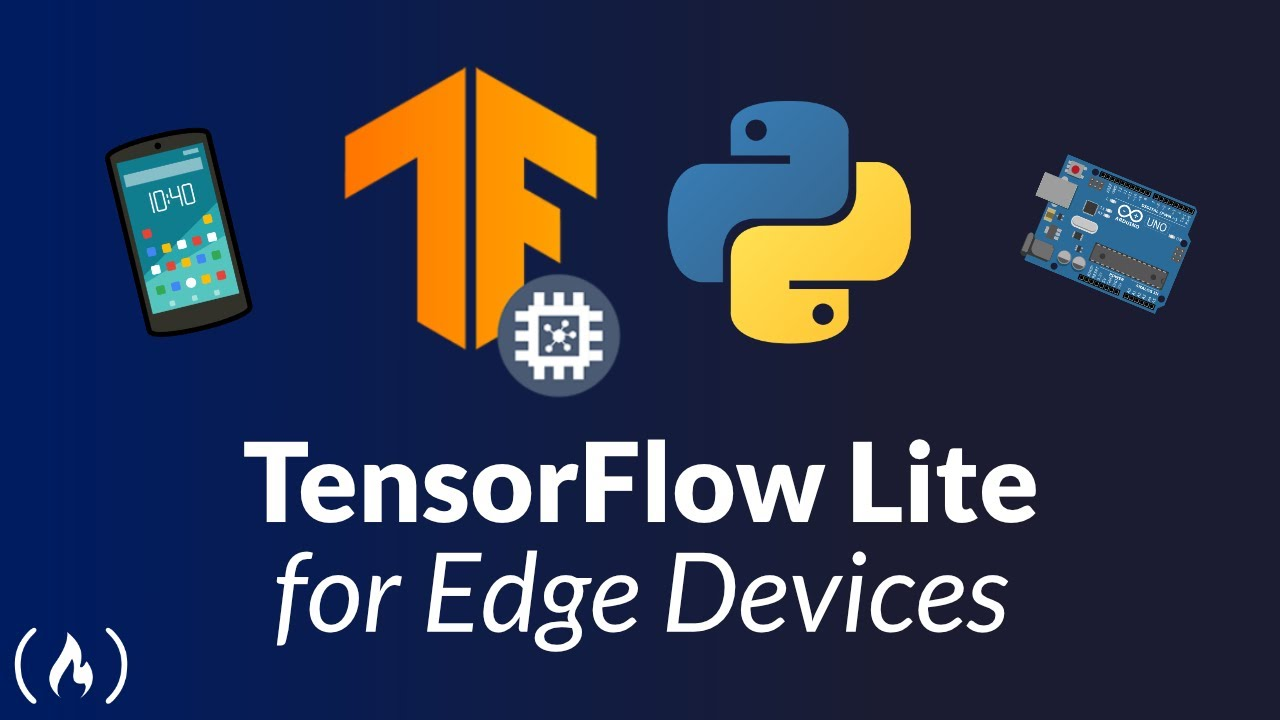# TensorFlow Lite for Edge Devices - TutorialLearn how to use TensorFlow Lite. TensorFlow Lite is an open source deep learning framework for on-device inference.

Learn how to use TensorFlow Lite. TensorFlow Lite is an open source deep learning framework for on-device inference.

⭐️ Course Contents ⭐️
(0:00:00) Introduction
(0:00:20) Why do we need TensorFlow Lite?
(0:04:10) What is Edge Computing?
(0:05:06) Why is Edge Computing gaining popularity?
(0:08:30) Challenges in deploying models on Edge devices
(0:09:27) What is TensorFlow Lite or TFLite?
(0:10:06) TensorFlow Lite Workflow
(0:13:30) Creating a TensorFlow or Keras model
(0:32:54) Converting a TensorFlow or Keras model to TFLite
(0:36:08) Validating the TFLite model performance
(0:46:08) What is Quantization?
(0:47:35) Compressing the TFLite model further
(0:51:55) Compressing the TFLite model even further
(0:55:08) Validating the most compressed TFLite model performance
(0:58:46) Thank You